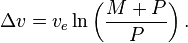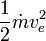# Rockets and kinetic energy or: Why do rockets (Space Shuttle) have rising accel?

So... there came a question up in another board which I can't answer... and perhaps you can give me an answer.

First of all there's the equation for kinetic energy: Ekin = 1/2 m v²

Which effectively means that the amount of energy needed for constant acceleration rises lineary to the velocity. Or: To accelerate from 0 to 1 m/s takes less energy than from 1 m/s to 2 m/s.

So far, so good. But unfortunately, spacecraft, such as the Space Shuttle, show a different behavior. Actually I'd expect a starting Shuttle to have the max G right after takeoff and acceleration dropping steadily from then on. Here's a chart I found and it shows that acceleration is steadily RISING during the second half of the flight:

http://www.russellwestbrook.com/launch.jpg [Broken]

Why?

A couple of ideas of mine:

- The longer the flight, the lighter the mass of the spacecraft, but since v² is the determining part of the equation, I wouldn't expect the mass change, unless it's somewhere in the vicinity of gigantic, to have such profound impact on the accel.
- Is perhaps the horizontal flight path at fault? During lift off, the Shuttle has to accelerate against earth's gravity, but when it's flying horizontally, thanks to centrifugal forces there's less to none gravity to compensate.

But I'm still not very sure. I hope you can help me solve this riddle.

Last edited by a moderator:

Spinnor
Gold Member
F = ma

The force is roughly constant with all engines working (I think they throttle back the main engines, the first dip in acceleration?, and then after the boosters seperate the main engines throttle back up) so as the mass constantly gets smaller acceleration generally increases.

rcgldr
Homework Helper
There's the equation for kinetic energy: Ekin = 1/2 m v²
Which effectively means that the amount of energy needed for constant acceleration rises lineary to the velocity.
In the case of a rocket, the total kinetic energy includes that of the spent fuel as well as the rocket. The total mechanical energy output is equal to the potential energy of the consumed fuel, minus losses to heat, and distributed between the spent fuel expelled from the rocket and the rocket itself. From an inertial frame of reference, as the rocket increases speed, more of the total mechanical energy goes into accelerating the rocket and less into accelerating the fuel. Wiki article:

http://en.wikipedia.org/wiki/Tsiolkovsky_rocket_equation

Note that the g force drops a bit below 1 g just after booster separation, then increases as the rocket gets lighter. The 3g peak at the end is because the engines are throttled back to reduce stress on the crew. Wiki article:

http://en.wikipedia.org/wiki/Space_shuttle#Launch

D H
Staff Emeritus
To accelerate from 0 to 1 m/s takes less energy than from 1 m/s to 2 m/s.
Playing devil's advocate: In a frame moving at 2 m/s relative to your first frame, the object starts with a velocity of 2 m/s, slows down to 1 m/s, and then down to 0 m/s. The change in kinetic energy from 2 m/s down to 1 is greater than that from 1 m/s to 0. That's exactly the opposite of what you wrote. So which view is correct?

The answer is neither one. There is no preferred frame of reference for a rocket. That is unlike the situation for a car, which gains or loses energy by directly interacting with the Earth.

Rockets gain velocity by ejecting mass at velocity. A rocket by itself does not obey conservation of energy. The rocket is not a closed system. Sans external influences such as gravity, energy is conserved by the system comprising the rocket plus the rocket's cloud of exhaust gases.

The easiest frame to use for analyzing a rocket's behavior is the rocket's instantaneous rest frame, the inertial frame that is instantaneously moving with the rocket. From here it is somewhat easy to derive the equation of motion for an ideal rocket in empty space, $\frac{d\vec v_r}{dt} = -\,\frac{\dot m_e}{m_r} \vec v_e$. This in turn leads to the ideal rocket equation.

A couple of ideas of mine:

- The longer the flight, the lighter the mass of the spacecraft, but since v² is the determining part of the equation, I wouldn't expect the mass change, unless it's somewhere in the vicinity of gigantic, to have such profound impact on the accel.
As noted above, v2 is not the determining part of the equation. The determining part of the equation is F=ma. If force stays the same, acceleration must necessarily increase as mass decreases.

You were however correct about the mass change. The mass change or a rocket such as the Shuttle is in vicinity of gigantic. A rocket that launches from the ground and goes into orbit loses 90% or so of its mass in 8 to 10 minutes. The mass change was the reason the Shuttle's acceleration increased as it climbed. The acceleration peaked at 3 g because the Shuttle's rockets were intentionally throttled back to keep acceleration from rising beyond 3 g.

A car accelerating away from us gains energy at 1/2mv2. As you clearly understand, this means that it's acceleration will slow down the faster it moves relative to the earth. This is of course true, as we move up the gears we have less torque at the wheels and acceleration drops off.

There are a few ways of understanding why this doesn't happen for a rocket. One that I like to use is that despite moving faster and faster away from the earth, a rocket doesn't actually push against it. It doesn't care whether it's standing still or moving 10000000 mph relative to the earth, sun, whatever, because the only thing it's pushing against is it's own fuel. In essence, a rocket takes its "earth", its frame of reference if you will, with it.

Its frame of reference is heavy, of course. As has been mentioned, it comprises more than 90% of the rocket's mass during lift-off, and hence the slow initial acceleration. In order to accelerate it constantly dumps it out the back to push against, thus losing considerable mass and gaining considerable acceleration.

Thanks for all answers, every one was very helpful. But I'm still sort of confused. I hope I got everything right.

The wikipedia link to the rocket equation article was very helpful. There was another link redirecting me to the Spacecraft Propulsion article. There I read about propulsive efficiency - the closer the rocket's and the exhaust's velocities are, the higher the propulsive efficiency is. Is this perhaps another part of the answer?

The solid rocket boosters exhaust velocity is somewhere around 2600 m/s. They are separated at ~3000 MPH / 1300 m/s which means that they go from under 10% efficiency to over 80% efficiency while the speed rises.
Then the main engines exhaust velocity is at 4400 m/s. Meaning that they'll start at over 50% efficiency and rise from then on, reach 100% at 10000 MPH and go down to ~85% from then on.

rcgldr
Homework Helper
I read about propulsive efficiency - the closer the rocket's and the exhaust's velocities are, the higher the propulsive efficiency is. Is this perhaps another part of the answer?
Propulsive efficiency considers how much of the total mechanical energy output goes into the rocket as opposed into the exhaust. For a given frame of reference where the rocket is going at the same speed as the exhaust speed relative to the rocket (but in opposite direction), then at that instant, the exhaust speed is zero, with zero energy, and all of the energy went into accelerating the rocket. Wiki article:

http://en.wikipedia.org/wiki/Propulsive_efficiency

Last edited:
Um, something different now:

(The question came up in a scifi-forum, so please don't bark at my insane numbers)

We do have a spaceship of 1'000'000 tons traveling at 500 km/s and then accelerating to 3100 km/s.
Let's assume the spaceship is using a nuclear fusion engine with an exhaust velocity of 30'000 km/s and and efficiency of 100%.

Using the ideal rocket equationI calculate that I need ~90'500 tons of propellant for a delta vee of 2600 km/s.

Now I'm calculating the kinetic energy of the exhaust withand get ~4.07 * 10^22 Joules.

But what about acceleration? Let's say I want a constant acceleration of ~150 G. Then, according to F = ma; thrust at the beginning of the flight would be at ~1.604 TN and at the end of the flight it would be ~1.471 TN (due to the lower mass).
And that's where my actual problem lies. Perhaps I have a wrong understanding of the connection between thrust, velocity gained and kinetic energy, but I'd think that one has to adjust the thrust (i.e. giving the engine "more power") on quite a large scale in order to hold a constant accel.
I think I'm having a rather gigantic knot in my brains, and hopefully you can help me unknot it.

rcgldr
Homework Helper
adjust the thrust on quite a large scale in order to hold a constant accel.
Look at the rocket equation again. As mass of the rocket and remaining on board fuel decreases, the amount of thrust required for constant acceleration decreases. Note that this is what happened near the end of the launch of the space shuttle, when the engine was throttled back in order to keep acceleration at 3 gs.

Very interesting graph (acceleration vs time) - one thing I do not understand though:

integrating the acceleration function with respect to time should amount to the total gain in velocity - which should be about 7800 m/s for a space shuttle in orbit. But the area under the graph doesnt amount to that...

help, anyone?

Best regards
torb

mfb
Mentor
I think the graph combines horizontal and vertical acceleration. In particular, it includes gravity (note that it is 1 before liftoff and drops in the roll maneuver).

At lift off, the Shuttle has only a little more thrust than weight, so it moves very slowly. The thrust of the solids, where most the mass is located is proportional to burn rate, so they are designed to burn much faster at first, then they slow down quite a bit after they dump much mass. At separation the total mass is a small fraction of what they started with. Then they speed up the liquid engines to 104% of their design point. That throttle up is the reason they only got one flight per overhaul instead of 25.